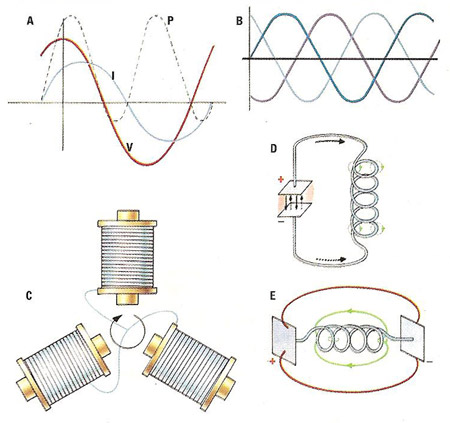A# alternating currentAlternating current is an electric current that changes direction periodically. The frequency of alternating current (AC) is measured in hertz (cycles per second). The frequency of the domestic supply is different in different parts of the world. In Europe, for example, it is 50 Hz (i.e. the current reverses direction 100 times per second), whereas in North America it is 60 Hz (current reversal 120 times a second).

Electrical energy can be produced by induction in a generator, a primary voltage causing an alternating current to flow in an external circuit. The presence of an inductor or capacitor (or both) produces phase displacement (A) between the voltage, V, and the current, I. Here a capacitor has produced a current phase lead of 90°, resulting in zero average power, although the power curve is still a sine wave. The reduction of power, P, due to the phase displacement, is called the power factor.

If three alternating currents have phase displacements of 120° relative to each other (see the accompanying diagram), the sum of their current or voltage is always zero (B). These three-phase currents are used in an electric motor with "squirrel cage" rotor (C). This consists of three electromagnets which rotate in the magnetic field produced.

Alternating currents are also produced in closed (D) and open (E) oscillating circuits. The high frequency electromagnetic waves used in some telecommunications originate from oscillating circuits.

The advantage of alternating current lies in the possibility of changing its voltage (i.e., to 110 V for domestic use or to higher voltages for long-distance transport).

An inverter can be used to convert from direct current (DC) to alternating current (AC). This is important for example, when a solar power system (producing direct current) needs to be connected to the grid or used for traditional home appliances,